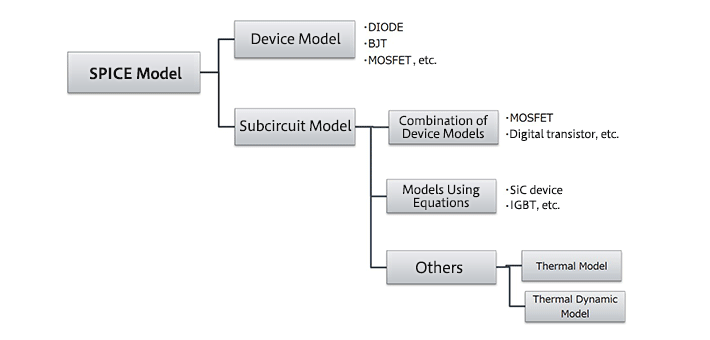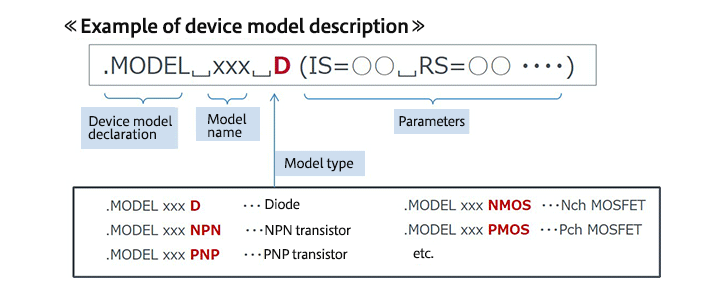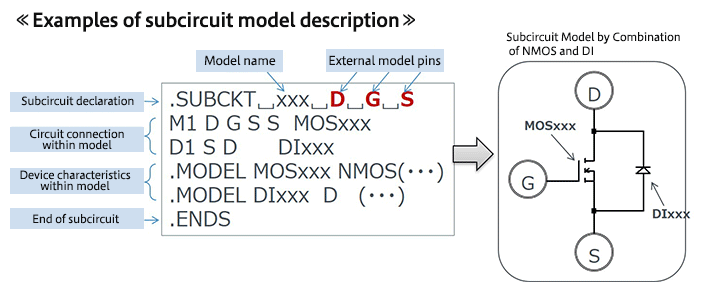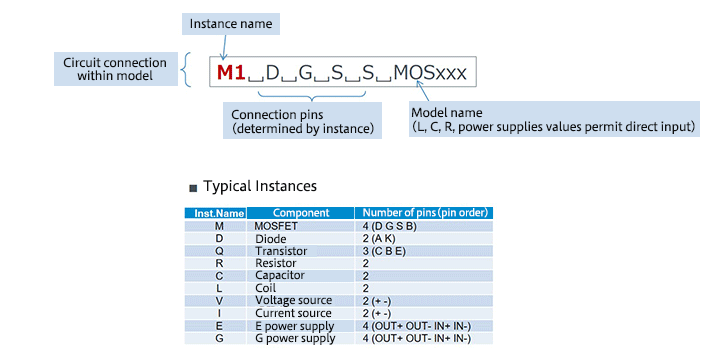Technical Information Site of Power Supply Design

2019.04.25 Simulation

# Types of SPICE Model

Foundations of Electronic Circuit Simulation

There are different kinds of models that are used in SPICE simulations. We have already explained a number of these, using the term "device models"; here we explain the types of SPICE model.

Types of SPICE Model

There are two types of SPICE model: "device models" and "subcircuit models".Device models essentially are models of elements such as diodes and MOSFETs. Subcircuit models are in essence combinations of device models, and can be thought of simply as circuit state models. In addition, there are also special models used to perform thermal calculations for circuits.

In device models, the electrical characteristics of the model, whether for a transistor, a diode, or another device, are determined by certain equations. The model indicates the coefficients (parameters) used in the equations.Device models, power supplies, equations, and so on are combined to configure a model as a circuit. The model includes circuit connection information, device models, and the like.Below are details of the "circuit connections within model" part of the above figure. An instance name is followed by connection pins and a model name.In the next article, we explain some models in somewhat greater detail.

#### Key Points:

・There are two types of SPICE models: “device models” and “subcircuit models”.

・Basically, ”device models” are models for discrete devices like transistors or diodes or like.

・”Subcircuit models” are basically a combination of “device models”.